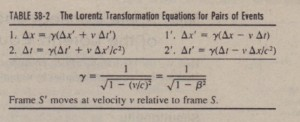# The Lorentz Transformation Equations

The Lorentz Transformation Equations

We state without proof that the correct transformation equations. which remain valid for all speeds up to the speed of light, can be derived from the postulates of relativity. The results, called the Lorentz transformation equations” or sometimes (more loosely) just ~he Lorentz transformations.(The equations are written with the assumption that 1 = I’ = 0 when the origins of Sand S’ coincide.) Note that the spatial values x and the temporal values 1are bound together in the first and last equations. This entanglement of space and time was a  prime message of Einstein’s theory. a message that was long rejected by many of his contemporaries.
It is a formal requirement of relativistic equations that they should reduce to familiar classical equations if we let (approach infinity. That is. if the speed of light were infinitely great, all finite speeds would be “low” and classical equations would You may wonder why we do Noi call these the EIII.’·Tcin transformation equations (and why not the Einstein factor for y). H. A. Lorentz actually denied these equations before Einstein did. Bui as the great Dutch physicist graciously conceded, he did not lake the further bold step of interpreting these equations as describing the true nature of space and lime. II IS this interpretation. first made by Einstein  that is at the hen of relativitynever fail. If we let c – 00 in Eqs. 38-20, “)’- I and these equations reduce-as we expect-to the Galilean equations (Eqs, 38-19), You should check this.  Equations 38-20 are written in a form that is useful if we are given x and t and wish to find x’ and t’, We may wish to go the other way, however. In that case we simply solve Eqs. 38-20 for x and t, obtaining

x = “)'(x’ + rr ‘) and t = “)'(1’ + n”‘/c2).

as measured by an observer in S, and

~x’ = x’; – x{ and ~t’ = t~ – t;.

as measured by an observer in S’, Table 38-2 displays the Lorentz equations in difference form, suitable for analyzing
pairs of events. The equations in   table were derived by simply substituting differences (such as ~x and ~x ‘) for the four variables in Eqs. 38-20 and 38-21. Be careful: When substituting values for these differences. you must be consistent  and not mix the values for the first event with those for the second event.Also, if. say. ~x is a negative quantity. you must be certain to include the minus sign in a substitution.